This page belongs to OCF Masters, a division of QC Avionix, LLC.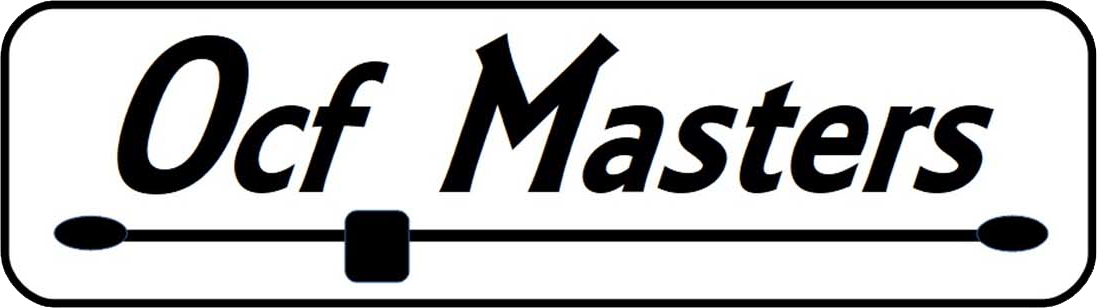# End Effect and Harmonic Antenna Design

Introduction

This article takes you through the design of a 40 M dipole that many hams also use on the third harmonic, 15 M. In the process it starts with the speed of light and drills down to the realities affecting our antennas including, Velocity Factor, VF, and End Effect in our battle to build multi-band harmonic antennas.

A 40 M Dipole

Let’s look at a 40 m half wave dipole with a center frequency of 7.15 MHz. A half wavelength, L, in free space is,

L = 0.5 * C/F = 0.5 * 299.7x108 / 7.15x106 ~ 300/F(MHz) = 20.98 m

in feet we have,

L=0.5* (300m/s / (0.3048m/ft */F) = 492/F = 492/7.15 ft = 68.8 ft

Where, C = the speed of light and F = frequency in MHz.

Simulating that in EZNEC in free space with #14 bare copper wire, we get the following: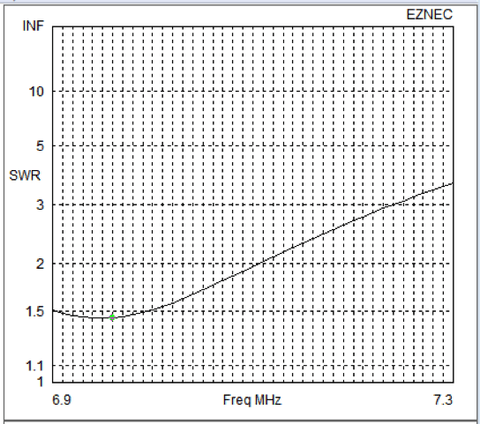The resonance, minimum reactance, occurs at 6.96 MHz instead of the expected 7.15 MHz and where does the familiar formula, L=486/F come in?  Why?

There are two electrical phenomena that cause the physical length of an antenna to be less than the electrical length, Velocity Factor (VF) and End Effect.

End Effect

Given that there is no wire beyond the end of the antenna, we expect the current at the end to be zero. To understand this, we model an antenna as a distributed collection of inductors, capacitors and resistors with a wave, our signal, propagating along its length. At the ends though the interaction of these elements is disturbed by the discontinuity at the end requiring the model of the end to have different equivalent circuit values. Namely, the inductance goes down and the capacitance goes up causing the voltage to go up at the ends.

Given that there is a high voltage at the ends of an antenna, we might suspect that there may be some conduction to objects that make up the other pole of the capacitor, and that is what happens as it reflects back towards the source creating a current flow from the ends of the wire. That current increases the electrical length of the antenna. How much the electrical length increases is a function of ratio of the length of the wire to its diameter called the K factor and is plotted below. (From ARRL Radio Amateur’s Handbook, 2007.)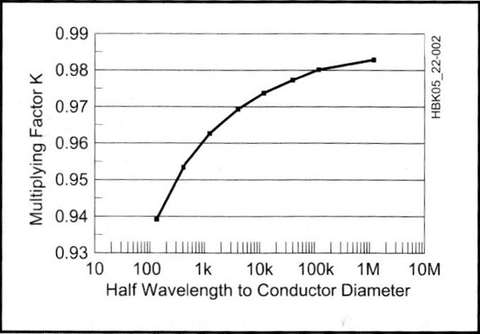Most wire antennas for HF have a L/D ratio greater than 10K. For our antenna the ratio is approximately K = 65*12/.064 = 13,000 and, from the chart, K = .975 and our formula becomes,

L = K*492/F = .975*492/F = 470/7.15 = 67.1 ft

The simulation in free space with bare copper wire below shows the resonance, minimum reactance, is 7.14MHz! If we use the formula we’ve all memorized for license exams for at least the last 70 years, L = 468/F, we get 65.45 ft. and resonance is out of the band, 7.31 MHz.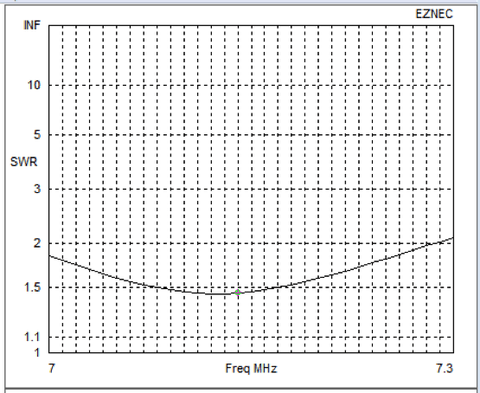Velocity Factor

Velocity factor is the ratio of the speed of propagation on the wire divided by the speed of light. The amount propagation is slowed depends on the physical properties of the antenna material, for our 40M antenna it’s wire. Bare copper wire doesn’t slow propagation and has a VF=1. However, insulation does affect propagation by adding a dielectric discontinuity and changing the effective diameter of the wire. The wire many hams use, THHN #14, has PVC insulation with a Dielectric Constant of 3.5 and insulation is .02 inches thick is included in the EZNEC simulation in free space below.The resonant frequency, minimum reactance, is 7 MHz, the bottom of the 40 M band and we have to shorten the antenna to 65.5 ft to resonate at 7.15 MHz.

Environment and Resonance

We know that the high voltage and low current at the ends increase the electrical length of the antenna in free space, consider now the impact of the physical environment on resonance. High voltage will couple to objects around the antenna including the support structure, rope, insulator, and even the ground below it causing current in addition to that occurring in free space that will further increase the electrical length of the antenna.

These factors that differ at each installation affect current flow from the ends are why our perfect calculations and measurements don’t usually deliver the resonant frequency we expected after we launch our antenna. Hence, we have to trim the length of our antennas. One would expect the capacitance to ground to increase as the antenna is lowered, increasing the current flow at the ends making the antenna look longer and lowering the resonant frequency.

The change in the resonant frequency of a #14 insulated wire 40 M antenna at different heights in the following table explains why we have to tune our antenna to deal with the environment around it. From what we have learned so far, we can conclude it’s wise cut the wire for a new antenna a bit longer than we calculated.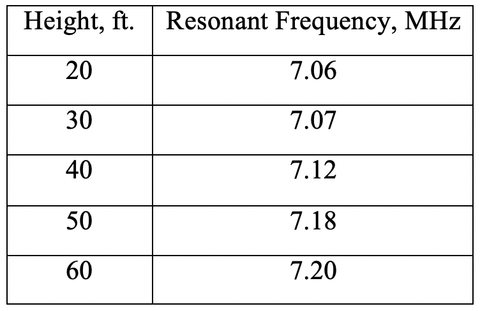Harmonic Resonance

It is well known that a 40 m dipole can be used on 15 m by operating on the third harmonic. We would expect the resonance to be 3 times the 40 m resonant frequency, 21.45 MHz. With 67.1 ft of bare wire in free space, the EZNEC simulation is shown below:Instead, we see that the resonance is 21.75 MHz. This anomaly can also be blamed on the end effect. Or, more precisely, the lack thereof. In third harmonic mode, the antenna looks like 3 co-linear dipoles placed end to end.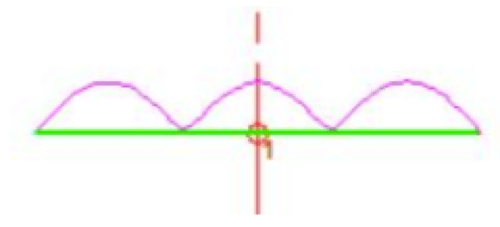The dipole half on the left end experiences End Effect on its left side while the dipole half on the right end experiences End Effect on its right side. Therefore, the length of the end halves is,

L(ends) = 470 / F

In the interior where the dipoles join, there is no End Effect and the L for the 2 internal dipoles is,

L(Middle) = 2*492 / F

And the total length needed to resonate on the third harmonic is,

L = 2*492 / F + 470 / F

The length for the middle of the 15 M band, 21.225 MHz is

L = (984+470) / 21.225 = 68.4 ft.

The free space bare wire SWR plot with resonance at 21.29 MHz is shown below.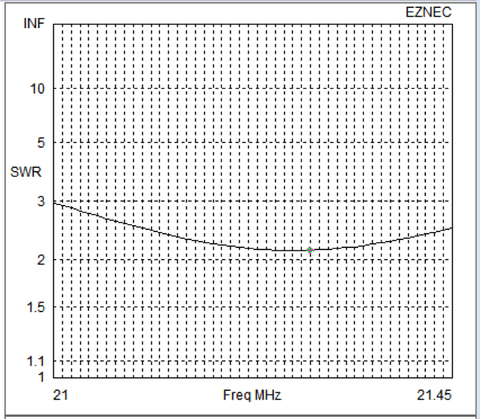We now know that if we put the antenna above ground the resonant frequency will be affected. More proof that antennas need to be adjusted to fit the environment.

We can generalize the results of End Effect for the nth harmonic as is especially useful for Off Center Fed antennas by recognizing that the two half wavelengths not on the ends don’t experience End Effect so they are each 492/F long opposed to the length of the two quarter waves at each end where the length is 470/F.

L=(n-1)*492 / F + 470 / F = ((n-1)*492+470)) / F

What length do we cut the antenna to make it resonate on both bands?

Cut for 7.15MHz the third harmonic is at 21.75 MHz, out of the band.

And, if we cut it for the middle of the 15 M band, 21.225 MHz,

the 40M resonance is below the band,

F = 470 / 68.5 = 6.86 MHz

Thus, the OCF designer’s conundrums:

1. If we cut it to place low SWR in middle of the lowest band, it will be too short for the higher bands. Conversely, if we cut the length to favor the high bands, it will be too long for the lower bands.
2. If we start by building the antenna for the middle of the lowest frequency band the harmonics quickly fall above the higher ham bands leaving us with major compromises. The last two columns in the table below show the results of starting with the higher bands giving us the opportunity to judiciously place capacitors to adjust resonance on the lower frequency bands as illustrated for our 40/15M dual band antenna below. Note that 20-6M lengths are less than 1 foot apart, only 0.3%.

This is illustrated below for lengths needed to achieve resonance for a 160M OCF by designing for the lowest frequency band vs. designing for higher frequency bands.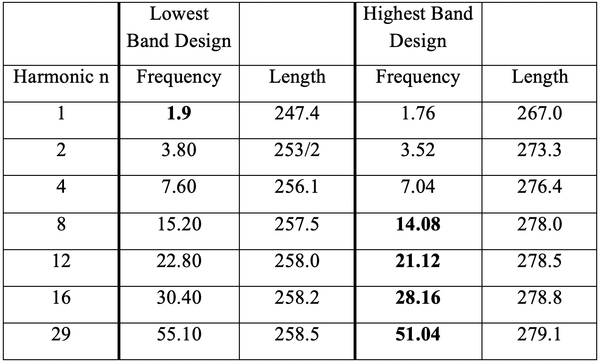Methods for Matching on BOTH Bands

The usual solution for dual band antennas is to start with an antenna designed for the lowest band place a resonant trap, parallel resonant circuit, tuned to the higher band on each leg to isolate the length tuned to the higher frequency from the lower frequency. The signal for the lower frequency passes through the trap giving a good match for the lower frequency while the trap isolates the longer wires from the higher frequency signal. The OCF Masters solution is simpler.

First tune the antenna to the higher frequency band. In this case, 15 M where the length is 68.5 ft. Then make the whole antenna resonant on both bands using one judicially place 390 pF capacitor, in this case one-third from the end at a current minimum on 15 M. This will only affect resonance on 40 M because no current flows through the capacitor on 15M. However, significant current flows there on 40M. The phase shift change of current flow on 40 M electrically shortens the antenna making it resonant on 40 M. Depending on the transmit power the capacitor may have to be rated at hundreds of volts and several amps. In this case at 1500 watts, 220 Volts and 3.6 Amps.

Before and After SWR plots are below.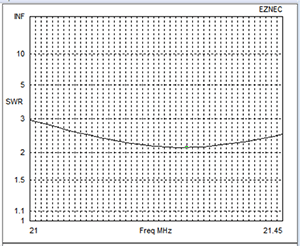15 M SWR Before Capacitor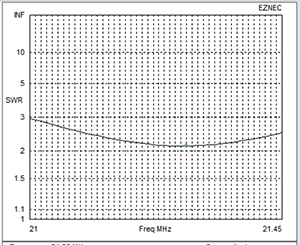15 M SWR After Capacitor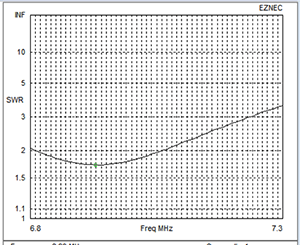40 M SWR Before Cap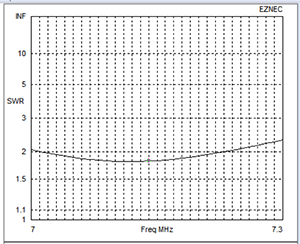40 M SWR After Capacitor

How did we get L = 468/F?

We discovered that 468/F isn’t correct for our antenna, so where did the number 468 come from? Despite extensive research, we’ve not found an explanation. Here is our story and we’re sticking to it until someone comes up with a better one.

Most wire antennas built in the early 20th century were not usually carefully tuned. Most were fed with open wire or even TV twin lead from broad range balanced tuners. (W1IS still has one he built in his shack) so resonance or SWR were never a serious consideration. Antennas were usually tuned by placing a neon bulb on or near the feed line or an RF ammeter was in the line between the transmitter and tuner. In fact, SWR bridges didn’t come into widespread use until the late 1950s with the advent of the Heathkit SWR bridge. Most hams had no idea what SWR their robust vacuum tubes rigs were seeing. However, in those days people were buying or building beams made from aluminum tubing with element diameters around one inch. The L/D ratio of a 20 M beam made from one inch tubing is,

L/D = 12*33/1 = 396 and from the K factor curve, K ~ 0.95,

yielding, L = 492*0.95 = 468

And that is our story of how we got the magic but incorrect number, 468, and we’re sticking to it until someone comes up with a better one. In fact, most of the authors then and now say that the classic formula is only a starting place.

Conclusion

We have used the design of a 40 M dipole to take a journey from the speed of light to the challenge in designing a multi-band harmonic antenna. Along the way we discovered that our “magic number” 468 isn’t really magic. It is a compromise to get us started as our antennas are really multi-dimensional and are affected by material, it’s size, height and location.

The Moral: Calculate carefully, add a foot or two to the wire you cut and be prepared to tune the antenna to resonate where you want it in your environment.

We also showed how our mismatched 40/15 M antenna can be matched on both bands using a single capacitor.